# blog posts# In this article, you will be fully acquainted with the different formats and types of variables in SPSS software to perform statistical tests with more accuracy, reliability and speed.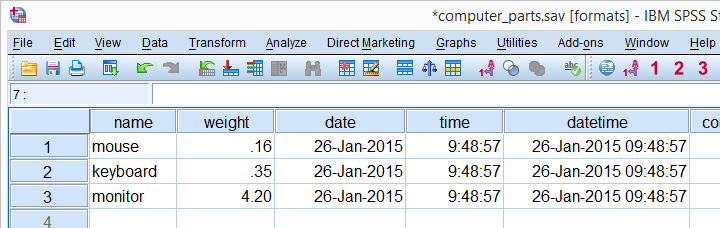## Variables in SPSS

SPSS software has two types of variables:

• Numerical variable : As the name implies, this variable contains only numbers and is mostly used in addition and multiplication calculations.
• String variable : This variable includes letters, numbers, and other characters. Even if string variables contain only numbers, they can still not be used to perform mathematical calculations.

SPSS software has no other variable in these two variables and other formats of numerical variables should not be confused with different types of variables in SPSS.

The best way to convert a string variable to a numeric variable (and vice versa) is to change the type of the variable. However, there are several other ways to do this that we will discuss below.

## Is a string variable better or a numerical variable?

One of the general rules in SPSS is:

In SPSS software, only nominal variables can be classified as string variables.

Names, email addresses, passport numbers and هستند are among the nominal variables. Although these variables give you useful information, they are usually not analyzed. Do not forget that numerical variables can be analyzed in specific cases such as nationality, blood type or occupation.

Quantitative string variables are different, for example the stringability of the dependent variable in analysis of variance (Anova) depends on the command you use, so you may be confused when working with quantitative string variables, so by converting the string variable to The numeric variable makes your job easier.

## Detect variable type in SPSS

Now how do we know if it is a numerical variable or a string? In version 24 and higher versions of SPSS software, there is a small icon next to the variable name that provides information about the type, format, and even measurement level of the variable. If the icon of the nominal variable contains the letter “a”, it means that it is a string variable. Note the following table: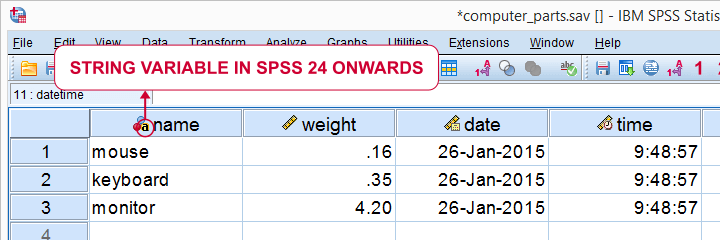In version 23 and lower versions of SPSS, use the following rule to detect a string and numeric variable:

• If the phrase “String” is written in the Type field, it is a String Variables.
• If the phrase “String” is not written in the Type field, it is a Numeric Variable.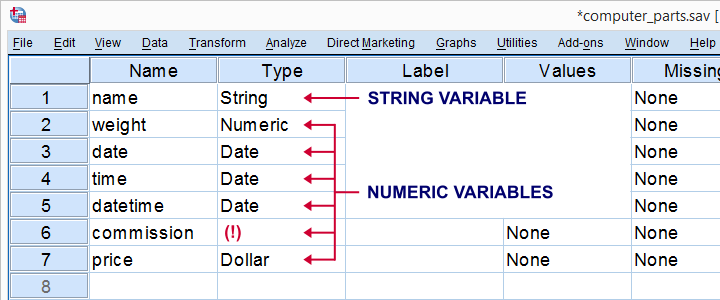Although Date and Dollar are variable formats, SPSS software incorrectly classifies the two as types of variables.

## Format variables in SPSS

First, take a look at the table below: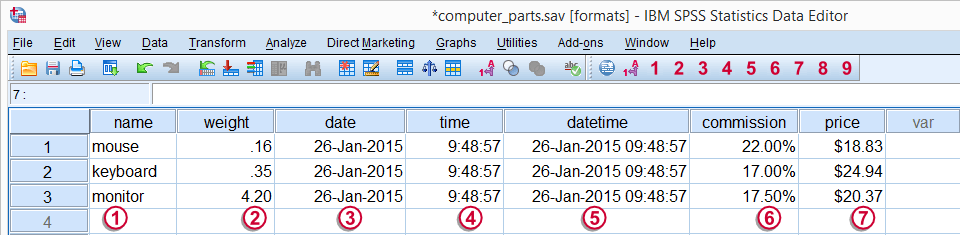According to the previous table, we know that variable number 1 is one of the string variables and variables number 2 to 7 are part of numerical variables.

Phrases like “26-Jan-2015” are considered SPSS formats.

## Detect variables in SPSS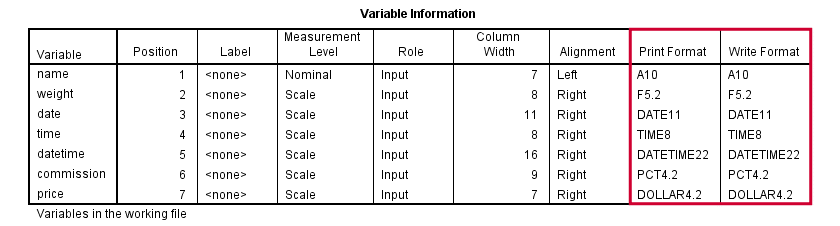In the Print / Write Format column of the table above you can see the different formats of the variables.

• The presence of the letter A at the beginning of the format means that it is a variable string.
• The letter F at the beginning of the format means that the variable is numeric.

The end numbers of the formats indicate the number of characters. The numbers after the dot show the number of numbers after the decimal point. Consider the following example: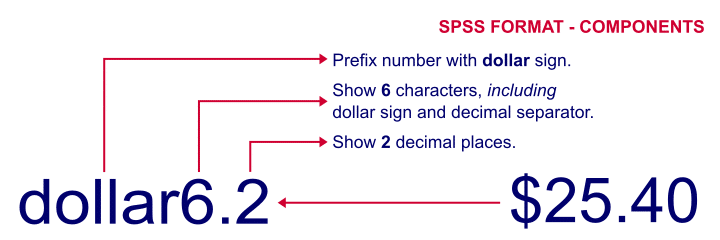The number 6 indicates the number of numbers and the number 2 indicates the number of numbers after the decimal point.

## Common Variable Formats in SPSS

Common string variable formats include A10, and common numeric variable formats include F5.2, Data11, and Time8.

## Adjust the format of variables in SPSS

The format of numeric variables can be changed by running a simple command. For example, to convert numeric variables to weight format, you must use the following command:

Weight format: 3. f4

Remember that changing the formatting of variables does not change your data. What you write in SPSS; It is different from what the software reads. For example, SPSS software reads \$ 20.37 as 20.37, and the dollar sign does not affect the result.

## Concluding remarks

Although the points mentioned in this tutorial were mostly theoretical, but with a little practice and repetition, you can become a professional in SPSS software in practice.